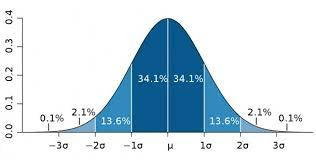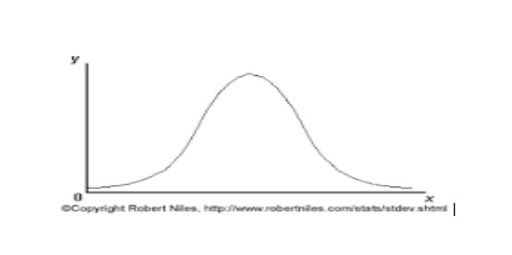# Standard Deviation and Bell Curves

APPLY NOW ???? WORK IN CANADA WITH FREE SPONSORSHIP!

What the graphical representation of standard deviation does is that it explains the whole set of data in a more elaborate way. In this article, we explain all about standard deviation and bell curves.

Standard deviation is one of the main statistical tools to find the dispersion of statistical data. The standard deviation calculator provides us with enough basis to make the bell curve of our statistical data.

When you are able to draw a bell curve then it is possible to figure out what the mean values are and what kind of dispersion we are dealing with in the whole dataset.

The sd calculator makes it easy to figure out the whole dispersion of the data, including the mean and the standard deviation in a matter of seconds.

You can tell what is the dispersion in our dataset values in our sample and in the population. This provides complete information regarding the target market.

The distribution of data is well represented by the bell shape graph, representing the symmetry and the distribution of the data. For making the bell shape first we need to find the frequency of all the dataset values.

This provides us the complete information about the data values.The Standard Deviation and Bell Curve

The mean identifies the mean position and the height of the bell curve. If the dispersion is larger a wider bell shape is going to be created showing the dispersion is greater in the whole set of the data.

Calculate standard deviation by the mean and standard deviation calculator and find what kind of dispersion you are dealing with to make the graphical representation possible for you.

On the other hand, the steeper and tall bell curve representing the dispersion is ever smaller and the whole set of the data is residing more closely to each other.

Let’s suppose an example:

5,6,8,11,14,12,11,15,13,14,11,5,6,9

The Frequency Table:

The standard deviation calculator uses the data 5,6,8,11,14,12,11,15,13,14,11,5,6,9 and generates the frequency table of all the values of the statistical data.

This data is utilized by the online tool to produce a bell-shaped graph of our statistical data. The bell graph actually enables us to understand the dispersion of the whole population.

This curve provides us he sufficient information regarding the whole population. The main purpose of the standards deviation is to extract the information from the data.

The frequency table of the data

Value Frequency 5 6 8 1 11 14 12 15 1 13 1 9 1

The frequency of 11 is the highest 3 and we have represented the whole set of data in the frequency table. The frequency of 5 is 2, the frequency of 6 is 2, 8,9, 15,13 has frequency is 1.

The 14 frequency has 2 along in the frequency table.

Standard Deviation and Bell Curves

The Coefficient of Variance:

The coefficient of variance is key to finding the standard deviation as it is, in this case, 0.35 and, and we have counted the number of members which is “14”, and the sum of all the values or the summation values is 140.

Now the variance of all the values and the standard error of the mean is 0.937.

Count (n)

14

Sum (Σx)

140

Mean (x̄)

10

Variance (s²)

12.31

Coefficient Of Variance

0.3508

Standard Error of Mean (SE)

0.93760588887717

The Standard Deviation is the Standard deviation calculated by the standard deviation calculation and it is around “3.5082”.

The data is entered in the standard deviation calculator, and we have to enter the data in the upper and the lower limit of the mean values.

Standard Deviation and Bell Curves

How to Make the Bell Shape Graph?

We need to evaluate the mean values, which in this case is “3.5082” and the lower and upper values. In this case, the lower and the upper values are 7 to the right and 7 to the left.

The standard deviation calculator assists to find all the values which we do need to include in the standard deviation calculation to find the deviation. Standard Deviation and Bell Curves.

The standard deviation calculator provides enough data to describe the bell graph of the standard deviation. The standard deviation is one of the main statistical tools for finding the whole nature of the population.

Standard Deviation and Bell Curves. You can predict the whole set of data responses by finding the standard deviation.What is Meant by the Bell Shape Graph?

There are certain meanings for the bell-shaped graph and it describes the various things about the standard deviation.

For example, if the bell-shaped graph is tightly close to each other it means the standard deviation has no larger dispersion, and the other thing is that

if the graph is wider then the dispersion is large and the data reside away from the mean values. The standard deviation is a statistical graph of how tightly the whole set of data is clustered around the mean values.

When the data is closely bonded to each other then the dataset makes a more stepper bell graph.

If the dispersion is spread throughout the data then the bell graph is horizontal in shape. Standard Deviation and Bell Curves

The bell shape is also describing the symmetry of the distribution of data.

The standard deviation calculator provides us with sufficient information regarding the whole population.

The business is always in pursuit of finding the demographics of the target market, the standard deviation can make it easy to understand the response of the whole population.

The businesses are going to invest millions of dollars in a certain market, and they want to dig for information that is actually near to reality.

The most realistic information about a certain market can make a brand more competitive in the marketplace. Standard Deviation and Bell Curves.

You can analyze the strength, weaknesses, opportunities, and threats to your competitors and also of your product and services. Standard Deviation and Bell Curves.

If this was helpful to you, you can share this with other scholars who may want to learn more about the standard deviation and bell curves.

CSN Team.

APPLY NOW ???? WORK IN CANADA WITH FREE SPONSORSHIP!

=> FOLLOW US ON INSTAGRAM | FACEBOOK & TWITTER FOR LATEST UPDATE